### Home > PC > Chapter 8 > Lesson 8.1.2 > Problem8-30

8-30.
1. Recall that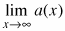= ∞ when a > 1. Find the following limits. Homework Help ✎

1., where a(x) = 3x

2.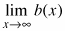, where b(x) = 3x − 6(2x)

3.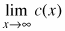, where c(x) = −5x

4.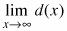, where d(x) = −5x + 10(4x)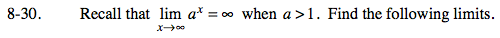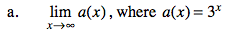Sketch a graph.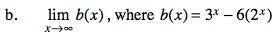What is the dominant term?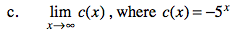Sketch a graph.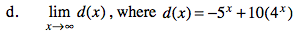What is the dominant term?

−5x